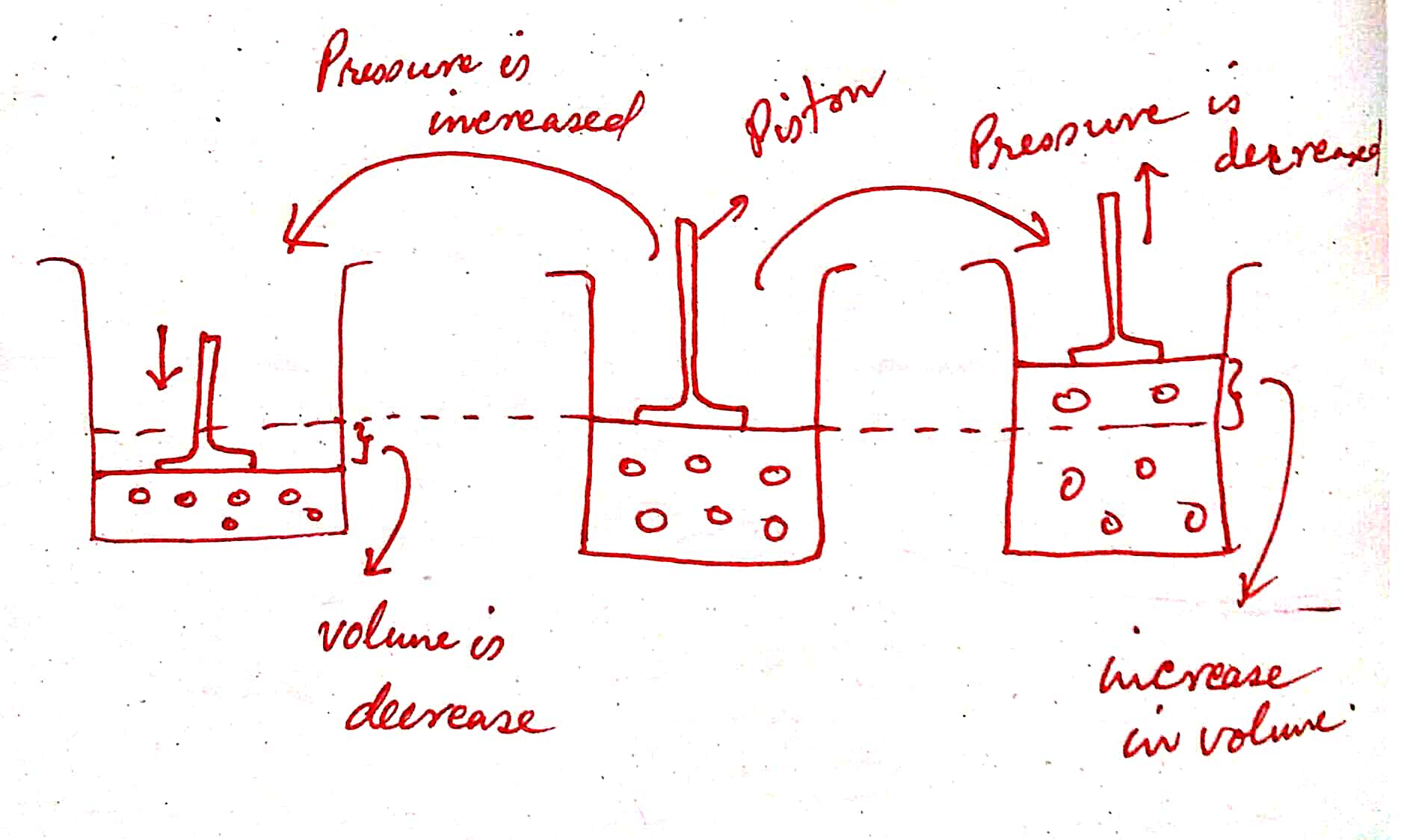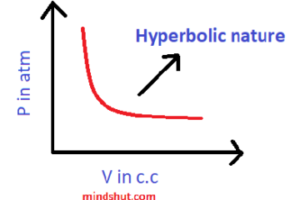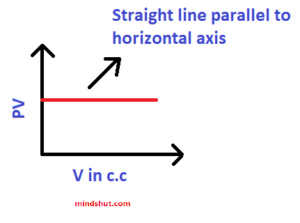# Boyle’s Law

The first modern chemist, Robert Boyle had proved and explained the two unique properties of any gas, expansibility, and compressibility.

#### Statement :

It states that  ” the volume of a given mass of a particular gas is inversely proportional to its pressure at a constant temperature.”

#### Explanation

Let us assume that a particular gas is enclosed in a closed container. Remember that the nature of the gas remains fixed throughout the experiment. Let us try to keep the temperature of the isolated system constant. After that, the piston is raised in an upward direction. As soon as the piston is raised, there is available more space. As we know that the gas molecules tend to occupy all the available space. In this way, the volume of the gas is increased.Now the piston is moved in a downward direction, all the molecules come to close each other. Hence, the volume of the gas decreased.

Thus we have a conclusion, that whenever the pressure increases the volume of the gas decreases and vice – versa but the temperature, mass and nature of the gas should be kept constant.

#### Constant Physical Quantities Involved in Boyle’s Law

1 ) Mass of the gas

2 ) The temperature of the gas

3 ) Number of molecules

#### Variable Physical Quantities Involved in Boyle’s Law

1 ) Volume of the gas

2 ) Pressure of the gas

#### Mathematical Expression

Let

P = Pressure of the gas

V = Volume of the gas

T = Temperature of the gas

M = Mass of the gas

From Boyle’s law, at a constant temperature,

V ∝ 1 / P

V = K / P

where K is proportionality constant.

PV = K

that is

P1 V1 = P2V2 = P3V3 =……………………………. = PnVn

This means that the product of the pressure and volume of the gas at constant temperature remains constant whatever be the value of pressure and volume.

#### Graphical Representation

https://youtu.be/Li2Lk2CDuho?list=PLY9WP9zM75IJjeSyOCO398Ou0ltPLBbv2

1 ) P – V graph:In the above graph, we may observe that the volume of the gas increases with a decrease in the pressure at constant temperature and vice – versa.

Nature of the graph → Hyperbolic nature

The shape of the graph → Hyperbolic shape

2 ) P – 1 / V graphNature of the graph  or shape of the graph → Straight line passing through the origin

3 ) PV – P graphAbove graph explains the following:

P1 V1 = P2V2 = P3V3 =……………………………. = PnVn

4 ) PV – V graphThis graph also explains the following

P1 V1 = P2V2 = P3V3 =……………………………. = PnVn

# Shorts Questions

1 ) State Boyle’s Law.

Ans :

It states that  ” the volume of a given mass of a particular gas is inversely proportional to its pressure at a constant temperature.”

2 ) Name the constants in Boyle’s Law.

Ans: Temperature, mass and no of moles

3  ) Which physical quantities are variable in Boyle’s Law?

Ans: Volume and pressure

4 ) What is the nature of the P-V graph?

Ans: Hyperbolic nature

5 ) Explain the PV = constant.

Ans: PV = Constant means that whatever be the values of pressure and volume, their product remains constant. That is, there is an indirect relation between the pressure and volume of the fixed mass of gas when its temperature remains constant. If the pressure increase, the volume of the fixed mass of the gas decrease as the molecules are compressed and on decreasing the pressure of the gas, the molecules have more and more space and hence its volume increases while the temperature should be kept constant.

6 ) Explain Boyle’s law.

Ans :

Let us assume that a particular gas is enclosed in a closed container. Remember that the nature of the gas remains fixed throughout the experiment. Let us try to keep the temperature of the isolated system constant. After that, the piston is raised in an upward direction. As soon as the piston is raised, there is available more space. As we know that the gas molecules tend to occupy all the available space. In this way, the volume of the gas is increased.Now the piston is moved in a downward direction, all the molecules come to close each other. Hence, the volume of the gas decreased.

Thus we have a conclusion, that whenever the pressure increases the volume of the gas decreases and vice – versa but the temperature, mass, and nature of the gas should be kept constant.

7 ) Write down the mathematical form of Boyle’s law and explain each term.

Ans :

Let

P = Pressure of the gas

V = Volume of the gas

T = Temperature of the gas

M = Mass of the gas

From Boyle’s law, at a constant temperature,

V ∝ 1 / P

V = K / P

where K is proportionality constant.

PV = K

that is

P1 V1 = P2V2 = P3V3 =……………………………. = PnVn

8 ) Give any two postulates of the kinetic theory of gas.

Ans :

i ) The gas molecules are point mass and have negligible volume.

ii ) The force of attraction or repulsion between the molecules of the gas is negligible.

9 ) Draw PV – V graph.

Ans :10 ) Draw the PV – P graph.

Ans :11 ) Draw P – V graph.

Ans :12 ) Draw P – 1 / V graph.

Ans :13 ) How does the volume vary with the pressure of the gas while the temperature of the gas remains constant?

Ans : The volume of the fixed mass of the gas varies inversely with the pressure while the temperature of the gas remains constant.

14 ) What is the relation between the pressure of the gas and its volume?

Ans : There is an in a direct relationship between the pressure and volume of the fixed mass of the gas at a constant temperature.

15 ) How does the increment of pressure affect the volume of the gas?

Ans : The increment of the pressure affects the volume of the fixed mass of the gas. At constant temperature, if the pressure increases the volume decreases and vice versa.

16 ) What is the SI unit of pressure?

Ans : The SI unit of pressure is Pascal.

17 ) What is the SI unit of volume?

Ans : The SI unit of volume is Cubic meter.

18 ) What is the CGS unit of pressure?

Ans : The CGS unit of pressure is dyne / squre cm

19 ) What is the CGS unit of volume?

Ans : The CGS unit of volume is cubic cm.

20 ) Name the instrument used to measure the atmospheric pressure?

Ans : Name of the instrument used to measure the atmospheric pressure is Manometer.

21 ) What is the value of normal pressure ?

Ans : 1 atm

22 ) What is the value of standard pressure?

Ans : 1 atm

23 ) What is the value of the standard temperature?

24 ) What is the value of the normal temperature ?

25 ) What is the meaning of STP?

Ans : STP means standard temperature and pressure.

26 ) What is the meaning of NTP?

Ans : NTP means normal temperature and pressure.

27 ) What is the value of 29 0 C in the Kelvin Scale?

Ans : 29 0 C =( 29 + 273 ) K = 303 K